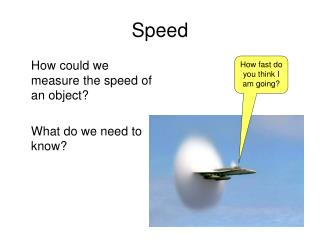DownloadDownload PresentationSpeed

# Speed

Download Presentation## Speed

- - - - - - - - - - - - - - - - - - - - - - - - - - - E N D - - - - - - - - - - - - - - - - - - - - - - - - - - -
##### Presentation Transcript

1. Speed How could we measure the speed of an object? What do we need to know? How fast do you think I am going?

2. Speed Speed = distance travelled time taken

3. Speed metres Speed = distance travelled time taken Metres per second (m/s) seconds

4. Speed kilometres Speed = distance travelled time taken Kilometres per hour (km/h) hours

5. Distance travelled? What if we know the speed of an object and the time it moved for and need to calculate the distance travelled?

6. Distance travelled? What if we know the speed of an object and the time it moved for and need to calculate the distance travelled? Speed = distance time distance = speed x time

7. Time? What if we know the speed and the distance travelled, how do we calculate how long (time) the journey took?

8. Time? What if we know the speed and the distance travelled, how do we calculate how long (time) the journey took? Speed = distance time time = distance speed

9. d s t x triangle

10. Don’t forget! If the distance is measured in metres and the time in seconds, -the speed will be in metres per second (m/s or m.s-1). If the distance is in kilometres and the time in hours, -the speed will be in kilometres per hour (km/h or km.h-1) etc.

11. Usian Bolt ran 100m in 9.58 seconds, what was his average speed? 10.43 m/s

12. Another example A dog runs at a speed of 5 m/s for one minute. How far does he run?

13. Another example A dog runs at a speed of 5 m/s for one minute. How far does he run? Distance = speed x time = 5 x 60 = 300 m

14. Another example! A car travels at 30 km/h and covers a distance of 900 km. How long did it take?

15. Another example! A car travels at 30 km/h and covers a distance of 900 km. How long did it take? Time = distance/speed = 900/30 = 30 hours

16. Now you try! • Extension: • How long does it take you to hop 5m? • Can you use a metre stick and stopwatch to help calculate your average hopping speed? • Also try: -walking backwards -hopping backwards